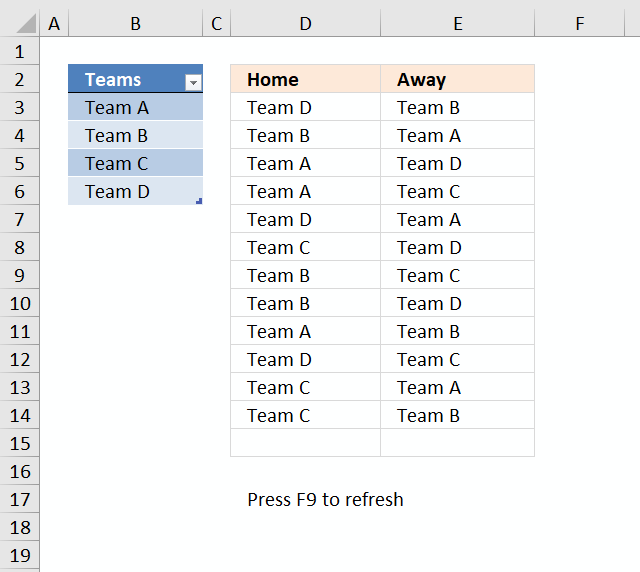Author: Oscar Cronquist Article last updated on January 03, 2019This article describes how to create a random playlist based on a given number of teams using an array formula. Column A contains four teams. Each team plays matches both "Home" against all remaining teams and "Away" against all remaining teams.

The teams are entered in an Excel defined table, this allows you to add or delete teams without the need to adjust cell references in the formulas. The calculation also takes into account the number of teams so the only thing for you to do is to extend the formulas down as far as needed.

Example:

Team A plays "Home" against Team B, Team C and Team D.

Team A- Team B
Team A - Team C
Team A - Team D

Team A plays also "Away" against Team B, Team C and Team D.

Team B - Team A
Team C - Team A
Team D - Team A

Column C and Column D contain a random playlist. Press F9 to refresh.

Array formula in D3:

=INDEX(Table1[Teams], LARGE((COUNTIF(\$D\$2:D2, Table1[Teams])<(COUNTA(Table1[Teams])-1))*ROW(\$1:\$4), RANDBETWEEN(1, SUMPRODUCT(--(COUNTIF(\$D\$2:D2, Table1[Teams])<(COUNTA(Table1[Teams])-1))))))

To enter an array formula, type the formula in a cell then press and hold CTRL + SHIFT simultaneously, now press Enter once. Release all keys.

The formula bar now shows the formula with a beginning and ending curly bracket telling you that you entered the formula successfully. Don't enter the curly brackets yourself.

If column A contains five teams, change ROW(\$1:\$4) to ROW(\$1:\$5) and bolded 3's to 4 in the above formula. Don't forget to adjust cell references.

Array formula i E3:

=INDEX(Table1[Teams], LARGE((COUNTIF(\$E\$2:E2, Table1[Teams])<(COUNTA(Table1[Teams])-1))*(COUNTIF(D3, Table1[Teams])=0)*(ISERROR(MATCH(Table1[Teams], IF(D3=\$D\$2:D2, \$E\$2:E2, ""), 0)))*ROW(\$A\$1:INDEX(\$A\$1:\$A\$1000, COUNTA(Table1[Teams]))), RANDBETWEEN(1, SUMPRODUCT(--((ISERROR(MATCH(Table1[Teams], IF(D3=\$D\$2:D2, \$E\$2:E2, ""), 0)))*(COUNTIF(\$E\$2:E2, Table1[Teams])<(COUNTA(Table1[Teams])-1))*(COUNTIF(D3, Table1[Teams])=0))))))

If column A contains five teams, change ROW(\$1:\$4) to ROW(\$1:\$5) and bolded 3's to 4 in the above formula. Don't forget to adjust cell references accordingly as well.

### Explaining formula in cell D3

There is an explanation to this formula in this post: Team generator

### Explaining formula in cell E3

#### Step 1 - Count previous teams in cells above

The COUNTIF function counts values based on a condition or criteria, the first argument contains an expanding cell reference, it grows when the cell is copied to cells below. This makes the formula aware of values displayed in cells above. 0 (zero) indicates values that not yet have been displayed.

COUNTIF(\$E\$2:E2,Table1[Teams])

becomes

COUNTIF(\$E\$2:E2,\$B\$3:\$B\$6)

becomes

COUNTIF("Away",{"Team A"; "Team B"; "Team C"; "Team D"})

and returns {0;0;0;0}.

#### Step 2 - Count non-empty cells

The COUNTA function counts cells that are not empty based on a given cell range.

COUNTA(Table1[Teams])-1

becomes

COUNTA(\$B\$3:\$B\$6)-1

becomes

COUNTA({"Team A"; "Team B"; "Team C"; "Team D"})-1

becomes

4-1

and returns 3.

#### Step 3 - Compare array with the number of teams - 1

COUNTIF(\$E\$2:E2, Table1[Teams])<(COUNTA(Table1[Teams])-1)

becomes

{0;0;0;0}<3

and returns

{TRUE; TRUE; TRUE; TRUE}

#### Step 4 - Prevent home team from being selected again

COUNTIF(D3,Table1[Teams])=0

becomes

COUNTIF("Team D",{"Team A"; "Team B"; "Team C"; "Team D"})=0

becomes

{0;0;0;1}=0

and returns

{TRUE; TRUE; TRUE; FALSE}

#### Step 5 - Prevent duplicate matches

This step makes sure that the same team is not being in the list more than once against a given Home team. There are two expanding cell references that grow when the cell is copied to cells below.

ISERROR(MATCH(Table1[Teams], IF(D3=\$D\$2:D2, \$E\$2:E2, ""), 0))

becomes

ISERROR(MATCH(Table1[Teams], IF("Team D"="Home", "Away", ""), 0))

becomes

ISERROR(MATCH(Table1[Teams], "", 0))

becomes

ISERROR(MATCH({"Team A"; "Team B"; "Team C"; "Team D"}, "", 0))

becomes

ISERROR({#VALUE!; #VALUE!; #VALUE!; #VALUE!})

and returns

{TRUE; TRUE; TRUE; TRUE}

#### Step 6 - Multiply arrays

This step applies and logic between the arrays meaning all conditions must be TRUE. TRUE * TRUE = TRUE, TRUE * FALSE = FALSE and FALSE * FALSE = FALSE. TRUE is 1 and FALSE is 0 (zero).

(COUNTIF(\$E\$2:E2,Table1[Teams])<(COUNTA(Table1[Teams])-1))*(COUNTIF(D3,Table1[Teams])=0)*(ISERROR(MATCH(Table1[Teams],IF(D3=\$D\$2:D2,\$E\$2:E2,""),0)))*ROW(\$1:\$4)

becomes

{TRUE; TRUE; TRUE; TRUE}* {TRUE; TRUE; TRUE; FALSE}* {TRUE; TRUE; TRUE; TRUE}* ROW(\$1:\$4)

becomes

{1; 1; 1; 0}* ROW(\$1:\$4)

The array is the multiplied to a sequence of row numbers.

{1; 1; 1; 0}* ROW(\$1:\$4)

becomes

{1; 1; 1; 0}* {1; 2; 3; 4}

and returns {1; 2; 3; 0}

#### Step 6 - Extract random row number

The LARGE function returns the k-th largest number, LARGE( array , k). The second argument k is a random number from 1 to n.

LARGE((COUNTIF(\$D\$2:D2, Table1[Teams])<(COUNTA(Table1[Teams])-1))*ROW(\$1:\$4), RANDBETWEEN(1, SUMPRODUCT(--((ISERROR(MATCH(Table1[Teams], IF(D3=\$D\$2:D2, \$E\$2:E2, ""), 0)))*(COUNTIF(\$E\$2:E2, Table1[Teams])<(COUNTA(Table1[Teams])-1))*(COUNTIF(D3, Table1[Teams])=0))))))

becomes

LARGE({1; 2; 3; 0}, RANDBETWEEN(1, SUMPRODUCT(--((ISERROR(MATCH(Table1[Teams], IF(D3=\$D\$2:D2, \$E\$2:E2, ""), 0)))*(COUNTIF(\$E\$2:E2, Table1[Teams])<(COUNTA(Table1[Teams])-1))*(COUNTIF(D3, Table1[Teams])=0)))))

The RANDBETWEEN function returns a random number based on the two arguments.

LARGE({1; 2; 3; 0}, RANDBETWEEN(1, SUMPRODUCT(--({TRUE; TRUE; TRUE; TRUE}*(COUNTIF(\$E\$2:E2, Table1[Teams])<(COUNTA(Table1[Teams])-1))*(COUNTIF(D3, Table1[Teams])=0)))))

becomes

LARGE({1; 2; 3; 0}, RANDBETWEEN(1, SUMPRODUCT(--({TRUE; TRUE; TRUE; TRUE}*{TRUE; TRUE; TRUE; TRUE}*(COUNTIF(D3, Table1[Teams])=0)))))

becomes

LARGE({1; 2; 3; 0}, RANDBETWEEN(1, SUMPRODUCT(--({TRUE; TRUE; TRUE; TRUE}*{TRUE; TRUE; TRUE; TRUE}*{TRUE; TRUE; TRUE; FALSE}))))

becomes

LARGE({1; 2; 3; 0}, RANDBETWEEN(1, SUMPRODUCT({1; 1; 1; 0})))

becomes

LARGE({1; 2; 3; 0}, RANDBETWEEN(1, 3))

becomes

LARGE({1; 2; 3; 0}, 2)

and returns 2. This is a random value between 1 and 3.

#### Step 7 - Return value

The INDEX function returns a value based on a cell reference and column/row numbers.

INDEX(Table1[Teams], LARGE((COUNTIF(\$E\$2:E2, Table1[Teams])<(COUNTA(Table1[Teams])-1))*(COUNTIF(D3, Table1[Teams])=0)*(ISERROR(MATCH(Table1[Teams], IF(D3=\$D\$2:D2, \$E\$2:E2, ""), 0)))*ROW(\$A\$1:INDEX(\$A\$1:\$A\$1000, COUNTA(Table1[Teams]))), RANDBETWEEN(1, SUMPRODUCT(--((ISERROR(MATCH(Table1[Teams], IF(D3=\$D\$2:D2, \$E\$2:E2, ""), 0)))*(COUNTIF(\$E\$2:E2, Table1[Teams])<(COUNTA(Table1[Teams])-1))*(COUNTIF(D3, Table1[Teams])=0))))))

becomes

INDEX(Table1[Teams], 2)

and returns "Team B" in cell D3.

### Get the Excel filerandom-plays.xlsx